# Square (geometry)

(diff) ← Older revision | Latest revision (diff) | Newer revision → (diff)
Square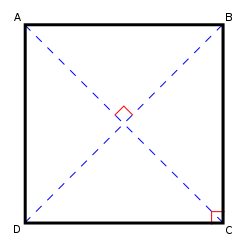A square
The sides of a square and its diagonals meet at right angles.
Edges and vertices 4
Schläfli symbols {4}
{}x{}
Coxeter–Dynkin diagrams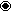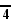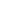Symmetry group Dihedral (D4)
Area
(with t=edge length)
t2
Internal angle
(degrees)
90°

In plane (Euclidean) geometry, a square is a regular polygon with four sides. It may also be thought of as a special case of a rectangle, as it has four right angles and parallel sides. Likewise, it is also a special case of a rhombus, kite, parallelogram, and trapezoid.

## Mensuration formulae

The perimeter of a square whose sides have length t is

$P=4t.$And the area is

$A=t^{2}.$In classical times, the second power was described in terms of the area of a square, as in the above formula. This led to the use of the term square to mean raising to the second power.

## Standard coordinates

The coordinates for the vertices of a square centered at the origin and with side length 2 are (±1, ±1), while the interior of the same consists of all points (x0, x1) with −1 < xi < 1.

## Properties

Each angle in a square is equal to 90 degrees, or a right angle.

The diagonals of a square are equal. Conversely, if the diagonals of a rhombus are equal, then that rhombus must be a square. The diagonals of a square are ${\sqrt {2}}$(about 1.41) times the length of a side of the square. This value, known as Pythagoras’ constant, was the first number proven to be irrational.

If a figure is both a rectangle (right angles) and a rhombus (equal edge lengths) then it is a square.

## Other facts

• If a circle is circumscribed around a square, the area of the circle is $\pi /2$(about 1.57) times the area of the square.
• If a circle is inscribed in the square, the area of the circle is $\pi /4$(about 0.79) times the area of the square.
• A square has a larger area than any other quadrilateral with the same perimeter.
• A square tiling is one of three regular tilings of the plane (the others are the equilateral triangle and the regular hexagon).
• The square is in two families of polytopes in two dimensions: hypercube and the cross polytope. The Schläfli symbol for the square is {4}.
• The square is a highly symmetric object. There are four lines of reflectional symmetry and it has rotational symmetry through 90°, 180° and 270°. Its symmetry group is the dihedral group $D_{4}$.
• If the area of a given square with side length S is multiplied by the area of a "unit triangle" (an equilateral triangle with side length of 1 unit), which is ${\tfrac {\sqrt {3}}{4}}$units squared, the new area is that of the equilateral triangle with side length S.

## Non-Euclidean geometry

In non-euclidean geometry, squares are more generally polygons with four equal sides and equal angles.

In spherical geometry, a square is a polygon whose edges are great circle arcs of equal distance, which meet at equal angles. Unlike the square of plane geometry, the angles of such a square are larger than a right angle.

In hyperbolic geometry, squares with right angles do not exist. Rather, squares in hyperbolic geometry have angles of less than right angles. Larger squares have smaller angles.

Examples: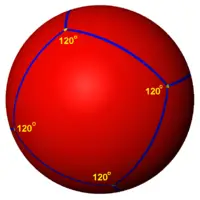Six squares can tile the sphere with three squares around each vertex and 120 degree internal angles. This is called a spherical cube. The Schläfli symbol is {4,3}.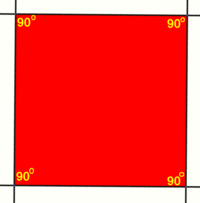Squares can tile the Euclidean plane with four around each vertex, with each square having an internal angle of 90 degrees. The Schläfli symbol is {4,4}.Squares can tile the hyperbolic plane with five around each vertex, with each square having 72 degree internal angles. The Schläfli symbol is {4,5}.